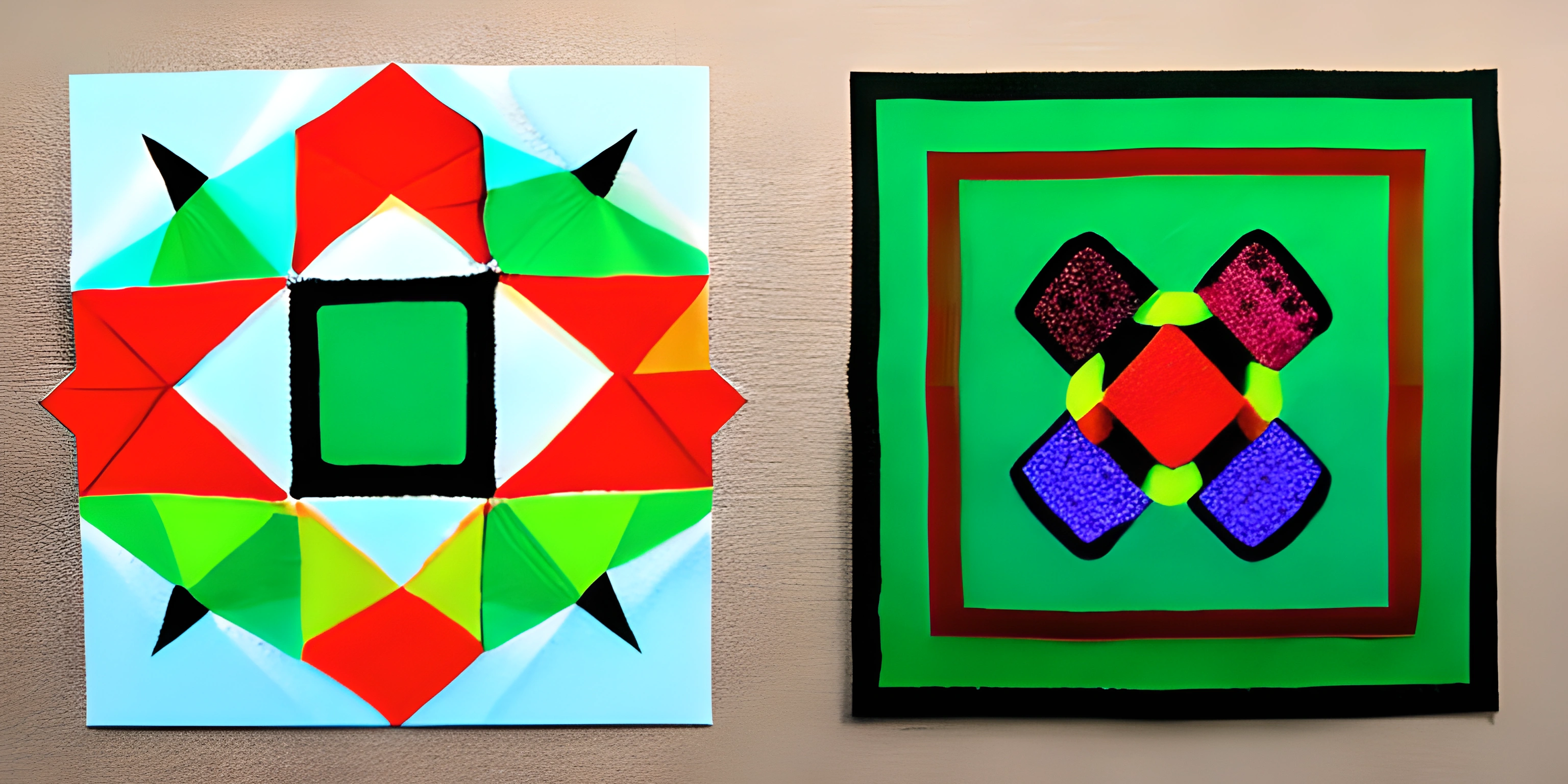# Creating Graphics with Python TurtleNote: this page has been created with the use of AI. Please take caution, and note that the content of this page does not necessarily reflect the opinion of Cratecode.

Creating graphics in Python can be a fun and visually rewarding way to learn programming. The Python Turtle library is an easy-to-use tool that allows us to create and manipulate graphics through a series of Turtle commands. So, let's dive into the world of Turtle graphics and unleash our creativity!

## What is Python Turtle?

Python Turtle is a built-in library that provides a simple way to create graphics and art using the turtle graphics system. The turtle graphics system originates from the Logo programming language, in which a small on-screen "turtle" acts as a cursor, drawing lines as it moves across the screen.

## Getting Started with Turtle

First, let's import the Turtle library and create a basic window to draw on. Remember, Turtle comes built-in with Python, so you don't need to install any additional packages.

``````import turtle

# Create a window to draw on
window = turtle.Screen()

# Create a turtle named "artist"
artist = turtle.Turtle()

# Draw a line
artist.forward(100)

# Close the window when clicked
window.exitonclick()``````

This simple program creates a window, initializes a Turtle object named `artist`, moves the turtle forward by 100 pixels to draw a line, and then waits for a click to close the window.

## Basic Turtle Commands

Now that we've created our first Turtle graphics program, let's explore some basic Turtle commands to control the turtle's movement, color, and shape.

### Movement Commands

• `forward(distance)`: Move the turtle forward by a specified distance.
• `backward(distance)`: Move the turtle backward by a specified distance.
• `right(angle)`: Turn the turtle to the right by a specified angle (in degrees).
• `left(angle)`: Turn the turtle to the left by a specified angle (in degrees).

### Color and Shape Commands

• `color(color_name)`: Set the turtle's pen color.
• `shape(shape_name)`: Set the turtle's shape (e.g., "turtle", "arrow", "circle", etc.).

## Example: Drawing a Square

Now, let's put those commands to use and draw a simple square.

``````import turtle

window = turtle.Screen()
artist = turtle.Turtle()

# Set the turtle's color and shape
artist.color("blue")
artist.shape("turtle")

# Draw a square
for _ in range(4):
artist.forward(100)
artist.right(90)

window.exitonclick()``````

Here, we've set the turtle's color to blue and its shape to resemble a turtle. Then, we use a loop to draw the square, moving forward 100 pixels and turning right by 90 degrees four times.

## More Complex Designs

With the basic Turtle commands under our belt, we can create more complex designs by combining loops, conditionals, and even incorporating user input. The possibilities are endless, so let your imagination run wild while you explore the world of Python Turtle graphics!

## FAQ

### What is Python Turtle Graphics?

Python Turtle Graphics is a library that allows you to create and manipulate graphics using the Turtle module in Python. It provides a simple, beginner-friendly way to draw shapes, create patterns, and explore programming concepts through visual feedback.

### How do I get started with Python Turtle Graphics?

To get started with Python Turtle Graphics, you first need to install the Turtle module, which is included in the standard Python library. You can start by importing the Turtle module using the following command:

``import turtle``

Then, create a new Turtle object to draw on the screen:

``my_turtle = turtle.Turtle()``

Now, you can use various Turtle methods to draw shapes and create graphics, such as `forward(distance)`, `right(angle)`, and `left(angle)`.

### Can you provide a basic example of Python Turtle Graphics?

Sure! Here's a simple example to draw a square using Python Turtle Graphics:

``````import turtle
# Create a Turtle object
my_turtle = turtle.Turtle()
# Draw a square
for i in range(4):
my_turtle.forward(100)
my_turtle.right(90)
# Close the Turtle graphics window
turtle.done()``````

### How do I change the color and pen size of my Turtle drawing?

To change the color and pen size of your Turtle drawing, you can use the `pencolor(color)` and `pensize(size)` methods of the Turtle object. Here's an example:

``````import turtle
my_turtle = turtle.Turtle()
# Set pen color to red and pen size to 5
my_turtle.pencolor("red")
my_turtle.pensize(5)
# Draw a square
for i in range(4):
my_turtle.forward(100)
my_turtle.right(90)
turtle.done()``````

### Can I create multiple Turtles in a single graphics window?

Yes, you can create multiple Turtle objects in a single graphics window. Each Turtle object will have its own properties and can be manipulated independently. Here's an example with two Turtles:

``````import turtle
# Create two Turtle objects
turtle1 = turtle.Turtle()
turtle2 = turtle.Turtle()
# Set properties for turtle1
turtle1.pencolor("blue")
turtle1.pensize(3)
# Set properties for turtle2
turtle2.pencolor("green")
turtle2.pensize(6)
turtle2.penup()
turtle2.goto(-100, 0)
turtle2.pendown()
# Draw squares with both turtles
for i in range(4):
turtle1.forward(100)
turtle1.right(90)
turtle2.forward(100)
turtle2.right(90)
turtle.done()``````